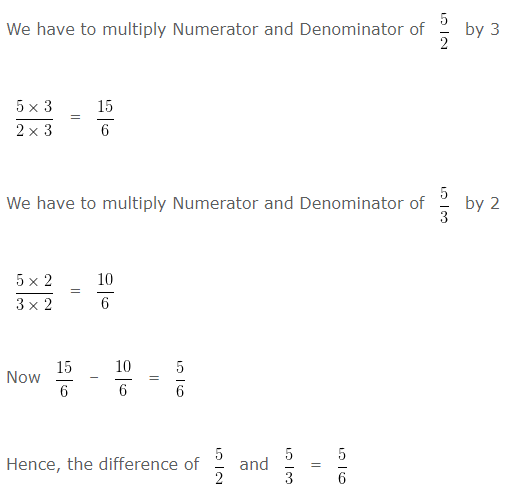# Subtracting Fractions

To subtract Fractions with same denominators ( Like Fractions ), we subtract the numerators and write the difference over the same denominator.

To subtract Fractions with different denominators ( Unlike Fractions ), first we have to change the Unlike Fractions, into equivalent Like Fractions, and then subtract the two equivalent Like Fractions

### Examples on Subtracting Fractions with like Denominators ( Like Fractions )

Example 1

Find the difference of 11/10 and 3/10 and reduce to its lowest term.

Solution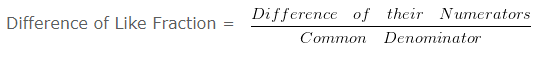= (11-3)/10

= 8/10

Simplifying further,
HCF of 8 and 10 is 2
Dividing both Numerator and Denominator by their HCF i.e,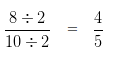Hence, the difference of 11/10 and 3/10 = 4/5

Example 2

Find the difference of 11/8 and 5/8 and reduce to its lowest term.

Solution= (11-5)/8

= 6/8

Simplifying further,
HCF of 6 and 8 is 2
Dividing both Numerator and Denominator by their HCF i.e,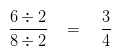Hence, the difference of 11/8 and 5/8 = 3/4

### Examples on Subtracting Fractions with Unlike Denominators ( Unlike Fractions )

Example 1

Find the difference of 13/6 and 4/3 and reduce to its lowest term.

Solution

To find the difference of Unlike Fraction

Firstly, we have to change the Unlike Fraction into equivalent Like Fraction, and then subtract the two equivalent Like Fraction

Take LCM of 6 and 3 is 6

Converting the Unlike Fraction into Equivalent Like Fraction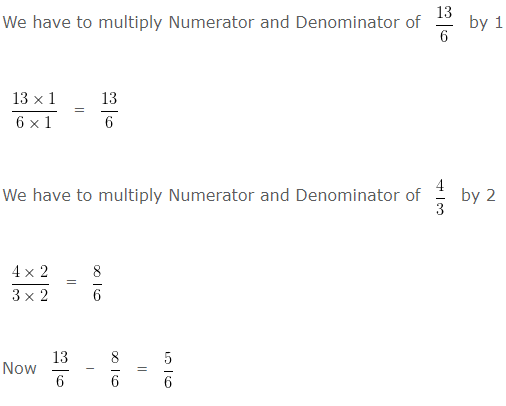Hence, the difference of 13/6 and 4/3 = 5/6

Example 2

Find the difference of 5/2 and 5/3 and reduce to its lowest term.

Solution

To find the difference of Unlike Fraction
Firstly, we have to change the Unlike Fraction into equivalent Like Fraction, and then subtract the two equivalent Like Fraction

Take LCM of 2 and 3 is 6

Converting the Unlike Fraction into Equivalent Like Fraction